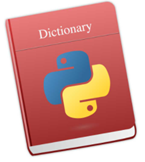## Thursday, October 22, 2020

### Python- How to Merge two DictionariesIf you are working as Python developer, data analytics or data scientists for any organisation then it is very important for you to know how to merge two dictionaries.

In Python, Dictionaries are written with curly brackets{}, and they are the combination of keys and values (key: value). The basic use of dictionaries in Python to store data values like a map, which unlike other Data Types that hold only single value as an element. Dictionaries is known as a collection which is unordered, changeable and indexed. If you knows about your keys in dictionaries then you can optimized it to retrieve values in a better ways.

Suppose that you have to add two dictionaries x and y in Python as given below -

`x = {'a': 1, 'b': 2}y = {'b':3, 'c':4}`
By creating a normal function — There is a very simple way to define a function in python by taking two dictionary variables as given below -
`def merge_two_dict(a,b):      # a local variable to copy the value from dict1      c= a.copy()      # update value in local variable from dict2      c.update(b)      # return merged value      return c`

Now, you have to call the above function to merge two dictionaries as given below -

`x = {'a': 1, 'b': 2}y = {'b':3, 'c':4} z = merge_two_dict(x, y)print(x){'a': 1, 'b': 2}print(y){'b': 3, 'c':4 }print(z){'a': 1, 'b': 3, 'c': 4}`

Efficient Way :Use lambda functionality — Some Python users think that lambdas are evil because lambda expressions are an odd and unfamiliar syntax to many Python programmers. But Lambdas are closures, which makes the space savings even bigger, since now you don’t have to add a parameterized constructor, fields, assignment code, etc to your replacement class.

You can write the fast and memory-efficient solution with one expression:

`x = {'a': 1, 'b': 2}y = {'b':3, 'c':4}# use lambda expressionsz = (lambda a, b: (lambda a_copy: a_copy.update(b) or a_copy)(a.copy()))(x, y)#print merged values in z variableprint(z){'a': 1, 'c': 4, 'b': 3}print(x){'a': 1, 'b': 2}print(y){'b': 3, 'c':4 }`
As suggested above, using two lines of code or writing a function is probably a better way to go.
http://www.sql-datatools.com